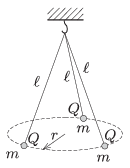Mathematical and Physical Journal
for High Schools
Issued by the MATFUND Foundation
 Already signed up? New to KöMaL?

#Problem P. 4656. (September 2014)

P. 4656. Three threads of equal length are hung at the same point and at the other end of each thread there is a small sphere, each having the same mass. The same charge is given to each of them. What is the radius of the circle at which the spheres are at rest?Data: $\displaystyle \ell=20$ cm, $\displaystyle m=1.3~$grams, $\displaystyle Q=1.2\cdot10^{-7}$ C.

(5 pont)

Deadline expired on October 10, 2014.

### Statistics:

 92 students sent a solution. 5 points: 52 students. 4 points: 21 students. 3 points: 15 students. 2 points: 3 students. 0 point: 1 student.

Problems in Physics of KöMaL, September 2014